# nrExtractResources

Extract resource elements from resource array

## Syntax

``re = nrExtractResources(ind,grid)``
``[re,reind] = nrExtractResources(ind,grid)``
``````[re1,...,reN,reind1,...,reindN] = nrExtractResources(ind,grid1,grid2,....,gridN)``````
``[___] = nrExtractResources(___,Name,Value)``

## Description

````re = nrExtractResources(ind,grid)` returns the resource elements from the resource array `grid` using resource element indices `ind`. The function can extract resource elements even if `grid` has a dimensionality which is different than the dimensionality of the indices `ind`. In this syntax, the specified indices are 1-based using linear indexing form.Typically, channel or signal specific functions generate resource element indices to map the channel or signal symbols to a resource grid. The indices address resource elements in an M-by-N-by-P array. M is the number of subcarriers, N is the number of OFDM symbols, and P is the number of antenna ports.For example, the following diagram highlights resource elements of a 4-by-4-by-2 resource array. The resource element indices are in 1-based linear indexing form. The number of the antenna ports is two (P = 2).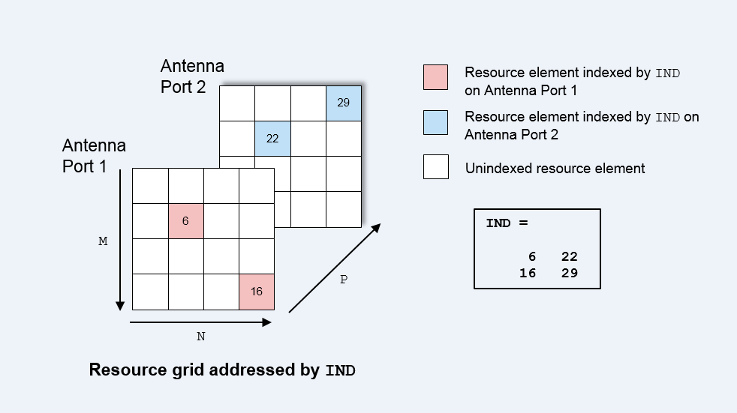```

example

````[re,reind] = nrExtractResources(ind,grid)` also returns `reind`, the indices of the extracted resource elements `re` within the resource array `grid`. The array `reind` is the same size as the extracted resource elements `re`.```
``````[re1,...,reN,reind1,...,reindN] = nrExtractResources(ind,grid1,grid2,....,gridN)``` extracts resource elements from multiple resource arrays using the resource element indices `ind`.```
````[___] = nrExtractResources(___,Name,Value)` specifies optional name-value pair arguments in addition to any of the input argument sets in previous syntaxes. Use these name-value pair arguments to specify the format of the input indices and the extraction method. Unspecified arguments take default values.```

## Examples

collapse all

Extract physical broadcast channel (PBCH) symbols from a received grid and associated channel estimates in preparation for decoding a beamformed PBCH.

PBCH Coding and Beamforming

Create a random sequence of binary values corresponding to a BCH codeword. The length of the codeword is 864, as specified in TS 38.212 Section 7.1.5. Using the codeword, create symbols and indices for a PBCH transmission. Specify the physical layer cell identity number.

```E = 864; cw = randi([0 1],E,1); ncellid = 17; v = 0; pbchTxSym = nrPBCH(cw,ncellid,v); pbchInd = nrPBCHIndices(ncellid);```

Use `nrExtractResources `to create indices for the two transmit antennas of a beamformed PBCH. Use these indices to map the beamformed PBCH into the transmitter resource array.

```carrier = nrCarrierConfig('NSizeGrid',20); P = 2; txGrid = nrResourceGrid(carrier,P); F = [1 1i]; [~,bfInd] = nrExtractResources(pbchInd,txGrid); txGrid(bfInd) = pbchTxSym*F;```

OFDM modulate the PBCH symbols mapped into the transmitter resource array.

`txWaveform = nrOFDMModulate(carrier,txGrid);`

PBCH Transmission and Decoding

Create and apply a channel matrix to the waveform. Receive the transmitted waveforms.

```R = 3; H = dftmtx(max([P R])); H = H(1:P,1:R); H = H/norm(H); rxWaveform = txWaveform*H;```

Create channel estimates including beamforming.

``` hEstGrid = repmat(permute(H.'*F.',[3 4 1 2]),[240 4]); nEst = 0;```

Demodulate the received waveform using orthogonal frequency division multiplexing (OFDM).

` rxGrid = nrOFDMDemodulate(carrier,rxWaveform);`

In preparation for PBCH decoding, extract symbols from the received grid and the channel estimate grid.

```[pbchRxSym,pbchHestSym] = nrExtractResources(pbchInd,rxGrid,hEstGrid); figure; plot(pbchRxSym,'o:'); title('Received PBCH Constellation'); ```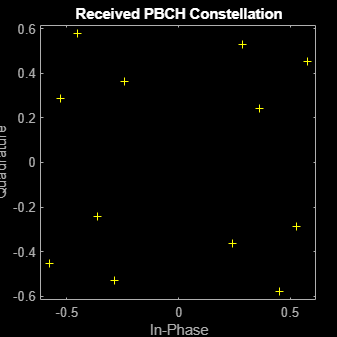Equalize the symbols by performing MMSE equalization on the extracted resources. Plot the results.

```pbchEqSym = nrEqualizeMMSE(pbchRxSym,pbchHestSym,nEst); figure; plot(pbchEqSym,'o:'); title('Equalized PBCH Constellation');```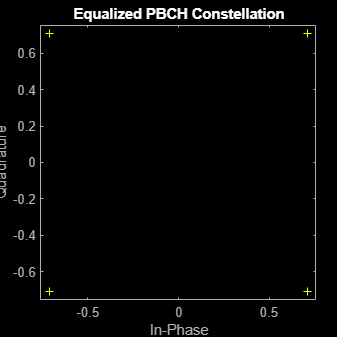Retrieve soft bits by performing PBCH decoding on the equalized symbols.

`pbchBits = nrPBCHDecode(pbchEqSym,ncellid,v)`
```pbchBits = 864×1 1010 × -2.0000 -2.0000 2.0000 -2.0000 -2.0000 2.0000 2.0000 -2.0000 -2.0000 -2.0000 ⋮ ```

## Input Arguments

collapse all

Resource element indices, specified as a matrix.

• If `'IndexStyle'` is `'index'`, each column of the matrix contains linear indices for the corresponding antenna.

• If `'IndexStyle'` is `'subscript'`, `ind` is a three-column matrix. The matrix rows correspond to the [subcarrier, symbol, antenna] subscripts based on the number of subcarriers, OFDM symbols, and antennas, respectively.

The function assumes that the indices are 1-based, unless you specify otherwise with the `'IndexBase'` argument.

Data Types: `double`

Resource array from which to extract resource elements, specified as one of these values:

• 3-D numeric array of size M-by-N-by-R that corresponds to a received grid — M is the number of subcarriers, N is the number of OFDM symbols, and R is the number of receive antennas. The grid is created after OFDM demodulation.

• A 4-D numeric array of size M-by-N-by-R-by-P that corresponds to a channel estimation grid — P is the number of antenna ports. The grid is created after channel estimation.

Data Types: `double`

### Name-Value Arguments

Specify optional comma-separated pairs of `Name,Value` arguments. `Name` is the argument name and `Value` is the corresponding value. `Name` must appear inside quotes. You can specify several name and value pair arguments in any order as `Name1,Value1,...,NameN,ValueN`.

Example: `nrExtractResources(ind,grid,'ExtractionMethod','direct','IndexBase','0based')` specifies direct extraction method with 0-based indexing.

RE indexing form, specified as one of these values:

• `'index'` — The indices are in linear index form.

• `'subscript'` — The indices are in [subcarrier, symbol, antenna] subscript row form.

Data Types: `char` | `string`

RE indexing base, specified as one of these values:

• `'1based'` — The index counting starts from 1.

• `'0based'` — The index counting starts from 0.

Data Types: `char` | `string`

Resource element extraction method, specified as the comma-separated pair consisting of `'ExtractionMethod'` and `'allplanes'` or `'direct'`.

• `'allplanes'` — The function extracts resource elements from each M-by-N plane within `grid`. The function uses indices that address unique subcarrier and symbol locations over all planes of the indexed resource array. See All-Planes Extraction Method (Default).

• `'direct'` — The function extracts resource elements from each M-by-N plane (for a 3-D `grid`) or M-by-N-by-R array (for a 4-D `grid`). The function uses indices that address the corresponding plane of the indexed resource array directly. See Direct Extraction Method.

Data Types: `string` | `char`

## Output Arguments

collapse all

Extracted resource elements, returned as a column vector, or a numeric array.

When `'ExtractionMethod'` is set to `'allplanes'`, the size of `re` is NRE-by-R-by-P, where:

• NRE is the number of resource elements extracted from each M-by-N plane of `grid`.

• R number of receive antennas.

• P is the number of planes.

When `'ExtractionMethod'` is set to `'direct'`, the size of `re` depends on the number of indices addressing each plane of the indexed resource grid.

• If the number of indices addressing each plane is the same, then `re` is of size NRE-by-R-by-P.

• If the number of indices addressing each plane is different, then `re` is a column vector containing all extracted resource elements.

For more details on the resource extraction methods, see Algorithms.

Indices of extracted resource elements within `grid`, returned as numeric array. `reind` is the same size as the extracted resource elements array `re`. The `reind` output inherits the indexing style and index base from `ind`.

## Algorithms

collapse all

### All-Planes Extraction Method (Default)

To use this method, set `'ExtractionMethod'` to `'allplanes'`. This method extracts resource elements from each M-by-N plane within `grid`. The indices address unique subcarrier and symbol locations over all the planes of the indexed resource array. The diagram highlights the indices used to extract resource elements from a resource grid with P = 2.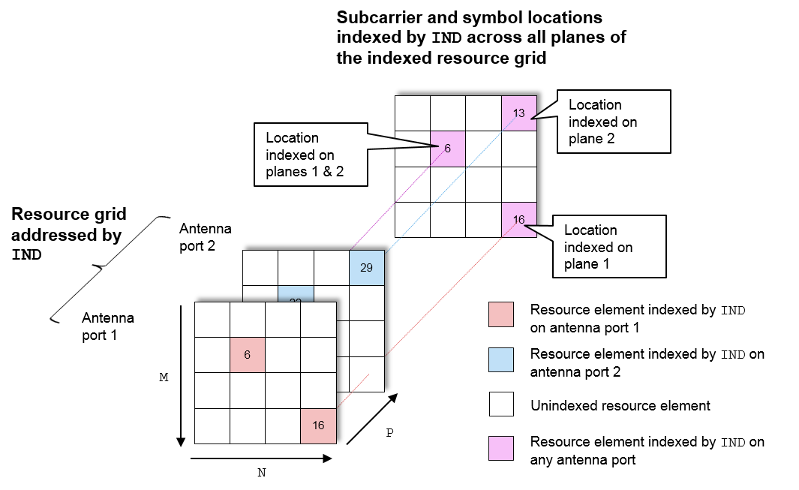Extraction Process for a 3-D Received Grid

The following diagrams illustrate the resource element extraction from a 3-D received grid, where the number of receive antennas R = 3. Resource elements are extracted from the grid at the symbol and subcarrier locations.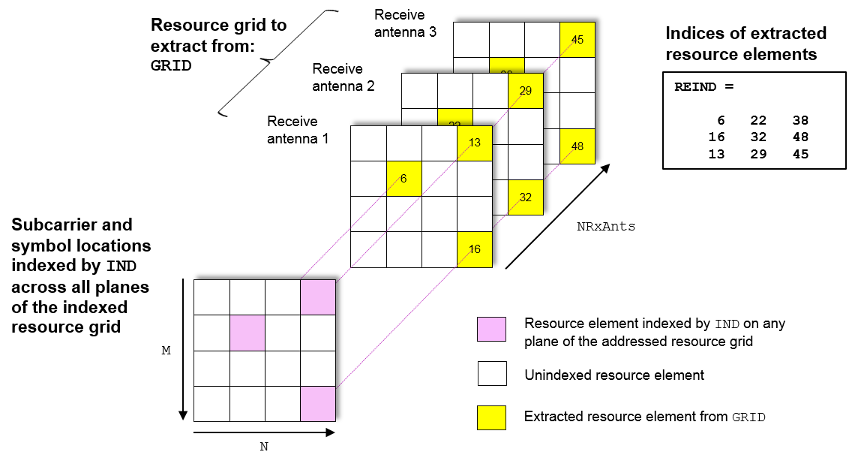Extraction Process for a 4-D Channel Estimate Grid

The following diagram shows the extraction process for a 4-D channel estimate grid. The number of receive antennas R = 3 and the number of antenna ports P = 2. The 4-D resource grid consists of P M-by-N-by-R arrays, each associated with an antenna port. Resource elements are extracted from all planes within these arrays.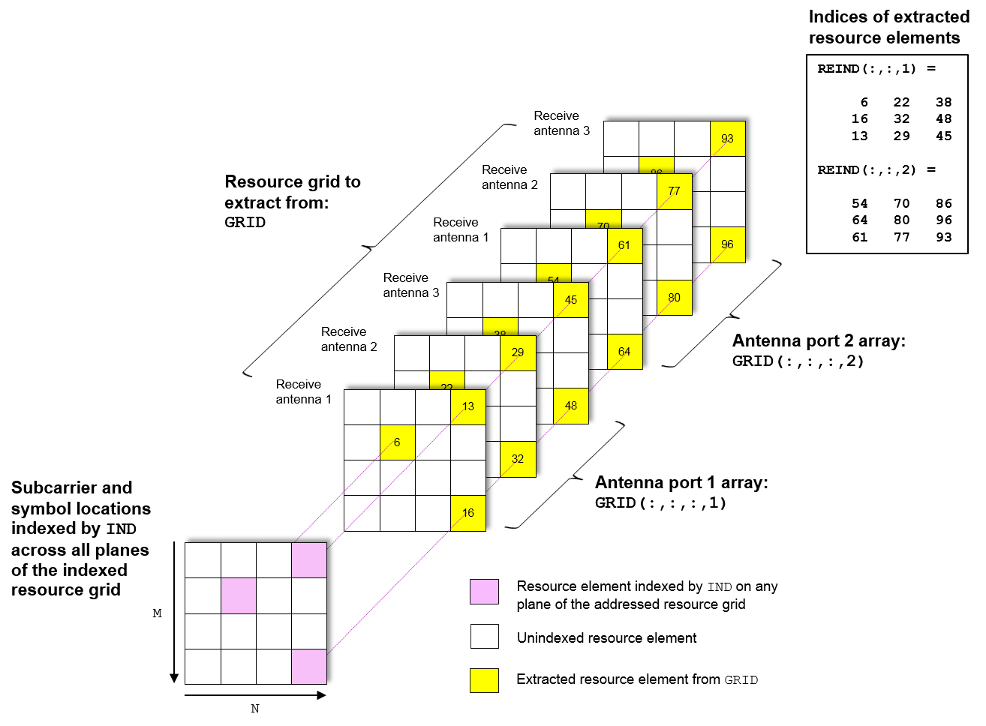### Direct Extraction Method

To use this method, set `'ExtractionMethod'` to `'direct'`. This method extracts resource elements from `grid` assuming that the third and fourth dimensions of the `grid` represent the same property as the planes of the indexed resource array such as antenna ports, layers, transmit antennas. Therefore the function extracts only the resource elements relevant to each plane of the indexed resource grid.

• For a 3-D `grid`, the direct method extracts elements from each M-by-N plane of `grid` using indices addressing the same plane of the indexed resource array. This method is the same as the standard MATLAB® operation `re` = `grid`(`ind`). Therefore, `reind` = `ind`.

• For a 4-D `grid`, the direct method extracts elements from each M-by-N-by-R array of `grid` by using indices addressing the same plane of the indexed resource array. The function assumes that the property represented by the planes of the indexed resource array is the same as the fourth dimension of `grid`.

Extraction Process for a 4-D Channel Estimate Grid

The following diagram shows the extraction process for a 4-D channel estimate grid. The number of receive antennas R = 3 and the number of antenna ports P = 2. The 4-D resource grid consists of P number of M-by-N-by-R arrays, each associated with an antenna port. The indices corresponding to each individual antenna port in the indexed resource array are used to extract resource elements from each of these arrays.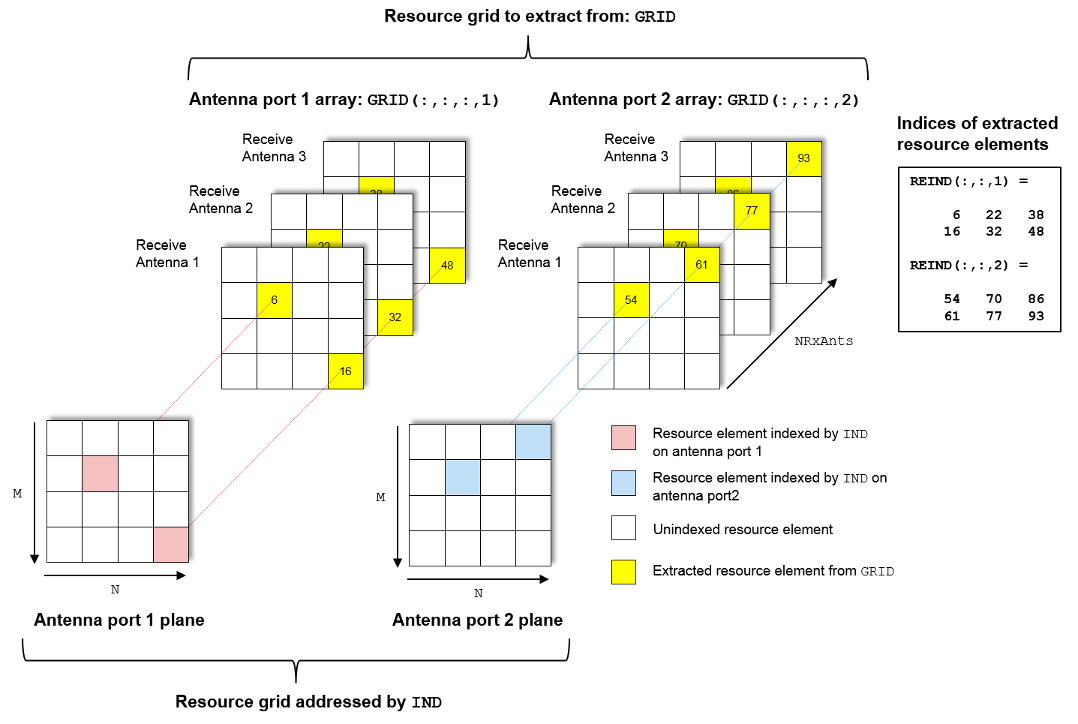## Extended Capabilities

Introduced in R2018b